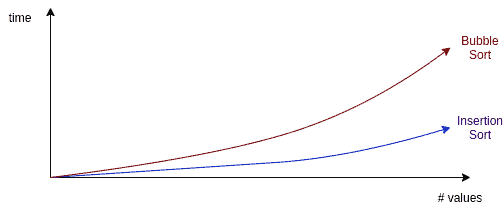## 1. Introduction

In this tutorial, we’ll learn about the similarities and the differences between insertion sort and bubble sort algorithms. We’ll compare these algorithms to understand their advantages and disadvantages better.

## 2. Overview

First of all, let’s remember the working principles of both algorithms.

### 2.1. Insertion Sort

As the name suggests, we insert the array elements into their proper positions one by one in insertion sort.

For theth iteration, the initialelements are sorted. We place theth element among the sorted part and extend it.

### 2.2. Bubble Sort

In bubble sort, we compare the adjacent elements and swap them when needed. After theth iteration, the lastelements are sorted.

## 3. Insertion Sort vs. Bubble Sort

First of all, let’s compare the basic properties of the algorithms:Both algorithms belong to the comparison sorting class. Hence, the place of each element is decided based on a comparison.

They are stable sorting algorithms. So, we don’t swap the keys having the same value during the sorting process. As a result, we preserve the initial ordering of such elements at the end.

The main difference between the algorithms lies in their method. Both of the algorithms compare the elements to find their order. Yet, onth iteration, the insertion sort algorithm compares theth element against the firstelements. On the contrary, on each iteration, the bubble sort algorithm compares and swaps the adjacent elements.

Both algorithms have a time complexity of. So, the time required to complete the sorting operation is quadratic:Similarly, the best and the average runtime complexities for both algorithms are the same.

Moreover, they both have a space complexity of. Thus, the extra space required by the algorithms to perform the sorting operation is not proportional to the input size. As both algorithms perform in place, this is an expected result.

In terms of complexity, both algorithms behave the same.

As we’ve already stated, the insertion sort and the bubble sort algorithms behave differently. For each iteration, insertion sort finds the proper place for theth element amongst the already sortedelements, situated at the beginning of the array. Conversely, bubble sort compares and swaps adjacent elements in each iteration:As a result, bubble sort performs more swap operations than the insertion sort. The high number of swaps leads to higher runtime for the bubble sort algorithm. Although both algorithms have the same complexity, the difference in runtime grows as the number of elements to be sorted increases on a random list:On average, the bubble sort performs poorly compared to the insertion sort. Due to the high number of swaps, it’s expected to generate twice as many write operations and twice as many cache misses. Therefore, we don’t prefer this algorithm for an ordinary sorting job.

Still, the bubble sort algorithm is favorable in computer graphics. It’s suitable for cases where we’re looking for a small error or when we have almost sorted input data.

All in all, insertion sort performs better in most cases. Consequently, it is more popular in textbooks and in real life.

## 4. Conclusion

In this article, we’ve compared two fundamental sorting algorithms: insertion sort and bubble sort.

They are both well-known comparison-based, in-place, stable sorting algorithms. Both algorithms are easy to understand and implement.

Both have similar runtime complexities. However, for larger datasets, the bubble sort algorithm becomes slower.